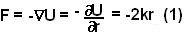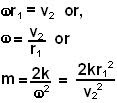## Saturday, June 28, 2008

### Irodov Problem 1.191

Force is the negative gradient of potential and so in polar coordinates we have,So essentially the force is a radial (central) force that is proportional to distance. As the particle moves in its orbit, the radial force must provide for the centripetal acceleration and so at any distance we have,Since m and k are constants, what eqn (2) indicates is that the angular velocity of the particle w will always be constant throughout its path. Since the particle's velocity v = wr. This further means that its distance from the center r and its velocity v will be inversely proportional. Hence, when the particle is the closest to the center (r=r1) is also the point when its velocity is the maximum (v2). In other words,#### 1 comment:Aenaith said...

Hello,
I find the step: "v = wr. This further means that its distance from the center r and its velocity v will be inversely proportional" a bit puzzling. Wouldn't v=wr mean that v and r are proportional?
Thanks :)
Amazing site btw :)